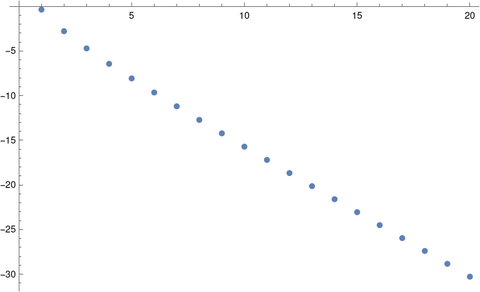# Approximating pi with Bernoulli numbers

In a paper on arXiv Simon Plouffe gives the formulawhich he derives from an equation in Abramowitz and Stegun (A&S).

It took a little while for me to understand what Plouffe intended. I don’t mean my remarks here to be criticism of the author but rather helpful hints for anyone else who might have difficulty reading the paper.

He says “Here the Bn are positive and n is even.” This doesn’t mean that the Bn are positive; it means that we should make them positive if necessary by taking the absolute value.

Plouffe says that he derives his estimate from an equation on page 809 of A&S, but at least in my copy of A&S, there are no equations on page 809. I believe he had in mind equation 23.2.16, which is on page 807 in my edition.

This equation saysThe first inequality in the paper does not appear directly in A&S but can be derived from the equation above. The approximation for π at the top of the post approximates ζ(2n) by 1. You could obtain a better approximation for π by using a better approximation for ζ(2n), which Plouffe does, retaining the first few terms in Euler’s product representation for the zeta function.

How good is the approximation above? Plotting the approximation error on a log scale gives nearly a straight line, i.e. the error decreases exponentially.This plot was produced with the following Mathematica code.

    f[m_] := (2 Factorial[2m] / Abs[BernoulliB[2m] 2^(2m)])^(1/(2m))
ListPlot[Table[Log[Abs[f[m] - Pi]], {m, 1, 20}]]


## 2 thoughts on “Approximating pi with Bernoulli numbers”

1. Steve Emmett

My version of A&S (AMS 55) also shows 23.2.16 on page 807.

2. Thanks. I didn’t think the book had changed since 1964, but I suppose there are minor differences in printings.

In any case, equation numbers are better than page numbers.